# Will I ever be a bayesian statistician ? (part 1)

Last week, during the workshop on Statistical Methods for Meteorology and Climate Change (here), I discovered how powerful bayesian techniques could be, and that there were more and more bayesian statisticians. So, if I was to fully understand applied statisticians in conferences and workshops, I really have to understand basics of bayesian statistics. I have published some time ago some posts on bayesian statistics applied to actuarial problems (here or there), but so far, I always thought that bayesian was a synonym for magician. To be honest, I am a Muggle, and I have not been trained as a bayesian. But I can be an opportunist…

So I decided to publish some posts on bayesian techniques, in order to prove that it is actually not that difficult to implement.As far as I understand it, in bayesian statistics, the parameter is considered as a random variable (which is also the case, in classical mathematical statistics). But here, here assume that this parameter does have a parametric distribution….
Consider a classical statistical problem: assume we have a sample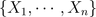i.i.d. with distribution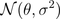. Here we note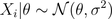since parameteris a random variable. The idea is to assume thathas a (so called a priori) distribution, e.g.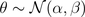So far it was simple. The idea is then to consider the posterior distribution of, given the observations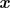. Thus, we need to compute the distribution of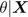which is here extremely simple (due to properties of the Gaussian distribution), i.e.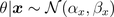where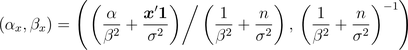And them, it becomes extremely natural to consider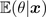as an estimator of given our sample data (and thus, we also have a confidence interval since we know the distribution ofgiven the observations).
In order to be sure that we understood, consider now a heads and tails problem, i.e.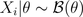. Note, first, that \theta has support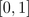. So we need a distribution on that support. Why not a beta distribution ? E.g.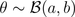Thus,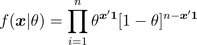and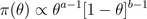From Bayes formula,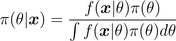and we get easily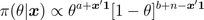which is the density of a Beta distribution, i.e.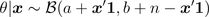prior=dbeta(u,a,b)
posterior=dbeta(u,a+y,n-y+b)

The estimator proposed is then the expected value of that conditional distribution,Note that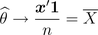Further, it is possible to derive confidence intervals using quantiles of the posterior distribution.
On the graphs below, we consider the following heads/tails sampleA first idea is to consider a uniform prior distribution.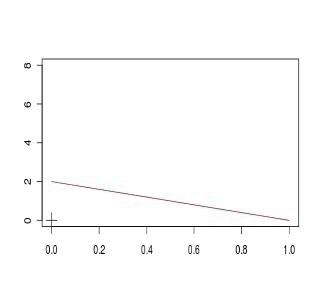A second idea is to consider an asymmetric beta distribution. First, with an asymmetry on the left,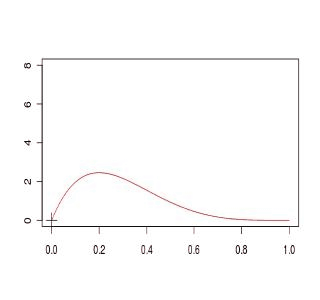or on the right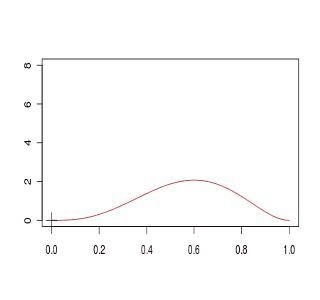Finally a third idea is simply to get back to the standard Gaussian approximation,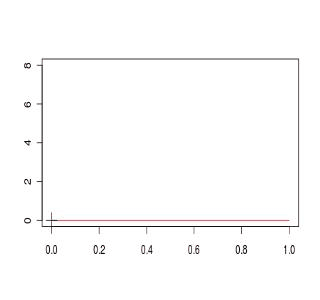If we compare the four models, we obtain (the plain black line is the Gaussian approximated distribution for the empirical mean), and red lines are obtained from prior beta distributionsThe code to generate those graphs is the following
a1=1; b1=1
D1[1,]=dbeta(u,a,b)
a2=4; b2=2
D2[1,]=dbeta(u,a,b)
a3=2; b3=4
D3[1,]=dbeta(u,a,b)
setseed(1)
S=sample(0:1,size=100,replace=TRUE)
COULEUR=rev(rainbow(120))
D1=D2=D3=D4=matrix(NA,101,length(u))
for(s in 1:100){
y=sum(S[1:s])
D1[s+1,]=dbeta(u,a1+y,s-y+b1)
D2[s+1,]=dbeta(u,a2+y,s-y+b2)
D3[s+1,]=dbeta(u,a3+y,s-y+b3)
D4[s+1,]=dnorm(u,y/s,sqrt(y/s*(1-y/s)/s))
plot(u,D1[1,],col="black",type="l",ylim=c(0,8),
xlab="",ylab="")
for(i in 1:s){lines(u,D1[1+i,],col=COULEUR[i])}
points(y/s,0,pch=3,cex=2)
plot(u,D2[1,],col="black",type="l",ylim=c(0,8),
xlab="",ylab="")
for(i in 1:s){lines(u,D2[1+i,],col=COULEUR[i])}
points(y/s,0,pch=3,cex=2)
plot(u,D3[1,],col="black",type="l",ylim=c(0,8),
xlab="",ylab="")
for(i in 1:s){lines(u,D3[1+i,],col=COULEUR[i])}
points(y/s,0,pch=3,cex=2)
plot(u,D4[1,],col="white",type="l",ylim=c(0,8),
xlab="",ylab="")
for(i in 1:s){lines(u,D4[1+i,],col=COULEUR[i])}
points(y/s,0,pch=3,cex=2)
plot(u,D4[s+1,],col="black",lwd=2,type="l",
ylim=c(0,8),xlab="",ylab="")
lines(u,D1[1+i,],col="blue")
lines(u,D2[1+i,],col="red")
lines(u,D3[1+i,],col="purple")
points(y/s,0,pch=3,cex=2)
}

Here, we can see that computations are simple if the prior distribution has a distribution which is the conjugate of the observations’ distribution (see here for the list of prior and posterior standard distributions).
So far, I have two questions that naturally show up

• is it possible to start with a neutral prior distribution, non informative ?
• what if we are no longer working with conjugate distributions ?

Well, I guess I have to work a bit more to answer those questions…. to be continued# Optimization and mixture estimation

Recently, one of my students asked me about optimization routines in R. He told me he that R performed well on the estimation of a time series model with different regimes, while he had trouble with a (simple) GARCH process, and he was wondering if R was good in optimization routines. Actually, I always thought that mixtures (and regimes) was something difficult to estimate, so I was a bit surprised…Indeed, it reminded me some trouble I experienced once, while I was talking about maximum likelihooh estimation, for non standard distribution, i.e. when optimization had to be done on the log likelihood function. And even when generating nice samples, giving appropriate initial values (actually the true value used in random generation), each time I tried to optimize my log likelihood, it failed. So I decided to play a little bit with standard optimization functions, to see which one performed better when trying to estimate mixture parameter (from a mixture based sample). Here, I generate a mixture of two gaussian distributions, and I would like to see how different the mean should be to have a high probability to estimate properly the parameters of the mixture.The density is hereproportional toThe true model is, andbeing a parameter that will change, from 0 to 4.
The log likelihood (actually, I add a minus since most of the optimization functions actually minimize functions) is
> logvraineg <- function(param, obs) {
+ p <- param
+ m1 <- param
+ sd1 <- param
+ m2 <- param
+  sd2 <- param
+  -sum(log(p * dnorm(x = obs, mean = m1, sd = sd1) + (1 – p) *
+ dnorm(x = obs, mean = m2, sd = sd2)))
+  }
The code to generate my samples is the following,
>X1 = rnorm(n,0,1)
> X20 = rnorm(n,0,1)
> Z  = sample(c(1,2,2),size=n,replace=TRUE)
> X2=m+X20
> X = c(X1[Z==1],X2[Z==2])
Then I use two functions to optimize my log likelihood, with identical intial values,
> O1=nlm(f = logvraineg, p = c(.5, mean(X)-sd(X)/5, sd(X), mean(X)+sd(X)/5, sd(X)), obs = X)
> logvrainegX <- function(param) {logvraineg(param,X)}
> O2=optim( par = c(.5, mean(X)-sd(X)/5, sd(X), mean(X)+sd(X)/5, sd(X)),
+   fn = logvrainegX)
Actually, since I might have identification problems, I take eitheror, depending whetheroris the smallest parameter.On the graph above, the x-axis is the difference between means of the mixture (as on the animated grap above). Then, the red point is the median of estimated parameter I have (here), and I have included something that can be interpreted as a confidence interval, i.e. where I have been in 90% of my scenarios: theblack vertical segments. Obviously, when the sample is not enough heterogeneous (i.e.andrather different), I cannot estimate properly my parameters, I might even have a probability that exceed 1 (I did not add any constraint). The blue plain horizontal line is the true value of the parameter, while the blue dotted horizontal line is the initial value of the parameter in the optimization algorithm (I started assuming that the mixture probability was around 0.2).
The graph below is based on the second optimization routine (with identical  starting values, and of course on the same generated samples),(just to be honest, in many cases, it did not converge, so the loop stopped, and I had to run it again… so finally, my study is based on a bit less than 500 samples (times 15 since I considered several values for the mean of my second underlying distribution), with 200 generated observations from a mixture).
The graph below compares the two (empty circles are the first algorithm, while plain circles the second one),On average, it is not so bad…. but the probability to be far away from the tru value is not small at all… except when the difference between the two means exceeds 3…
If I change starting values for the optimization algorithm (previously, I assumed that the mixture probability was 1/5, here I start from 1/2), we have the following graphwhich look like the previous one, except for small differences between the two underlying distributions (just as if initial values had not impact on the optimization, but it might come from the fact that the surface is nice, and we are not trapped in regions of local minimum).
Thus, I am far from being an expert in optimization routines in R (see here for further information), but so far, it looks like R is not doing so bad… and the two algorithm perform similarly (maybe the first one being a bit closer to the trueparameter).